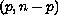Electron. J. Diff. Eqns., Vol. 2004(2004), No. 25, pp. 1-14.

### Multi point boundary-value problems at resonance for n-order differential equations: Positive and monotone solutions Panos K. Palamides

Abstract:
In this article, we study a complete-order differential equation subject to theright focal boundary conditions plus an additional nonlocal constrain. We establish sufficient conditions for the existence of a family of positive and monotone solutions at resonance. The emphasis in this paper is not only that the nonlinearity depends on all higher-order derivatives but mainly that the obtaining solution satisfies the above extra condition. Our approach is based on the Sperner's Lemma, proposing in this way an alternative to the classical methodologies based on fixed point or degree theory and results the introduction of a new set of quite natural hypothesis.

Submitted January 12, 2004. Published February 24, 2004.
Math Subject Classifications: 34B10, 34B18, 34B15, 34G20.
Key Words: Focal boundary value problem, multi-point, resonance, vector field, positive monotone solution, Sperner's lemma, Knaster-Kuratowski-Mazurkiewicz's principle.

Show me the PDF file (226K), TEX file, and other files for this article.Panos K. Palamides Naval Academy of Greece Piraeus, 185 39, Greece email: ppalam@otenet.gr   ppalam@snd.edu.gr http://ux.snd.edu.gr/~maths-ii/pagepala.htm